# Test Prep Plan - Take a practice test

Take this practice test to check your existing knowledge of the course material. We'll review your answers and create a Test Prep Plan for you based on your results.
How Test Prep Plans work
1
2Based on your results, we'll create a customized Test Prep Plan just for you!
3Study smarter
Study more effectively: skip concepts you already know and focus on what you still need to learn.

Exam Instructions:

Choose your answers to the questions and click 'Next' to see the next set of questions. You can skip questions if you would like and come back to them later with the yellow "Go To First Skipped Question" button. When you have completed the practice exam, a green submit button will appear. Click it to see your results. Good luck!

### Page 1

#### Question 1 1. Use the quadratic formula to find the solutions to the equation below.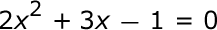#### Question 2 2. Find the value for c that must be added to both sides, and rewrite the equation in the vertex form.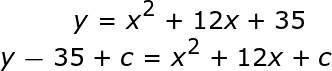#### Question 3 3. Find the solution to the equation below.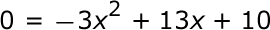#### Question 5 5. In order to complete the square on the following equation, what value would you add to both sides?### Page 2

#### Question 6 6. If the equation below told you a ball was 'h' feet in the air after 't' seconds, what would be the first step to finding out when it hit the ground?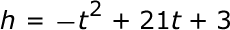#### Question 7 7. Find the roots of the equation.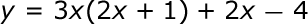#### Question 8 8. Find the solution to the equation below.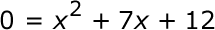#### Question 9 9. Find the roots of the equation below.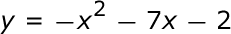### Page 3

#### Question 12 12. Rewrite the following equation in vertex form.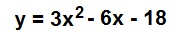#### Question 13 13. Rewrite the quadratic below in standard form: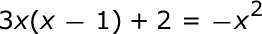#### Question 15 15. What would the first step be in completing the square of the following equation?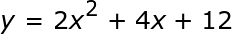### Page 4

#### Question 16 16. Solve the following equation.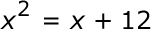#### Question 17 17. When completing the square for the quadratic equation shown below, how is c calculated?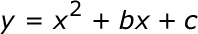#### Question 20 20. Expressing the equation below in the vertex form will give a value of 'h' = _____.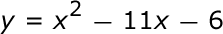### Page 5

#### Question 23 23. What is the b-value of the quadratic equation below?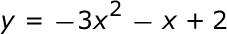### Page 6

#### Question 29 29. Which of the following are the solutions to the equation below?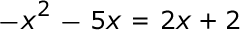#### Quadratic Equations and Functions: Business Math Lesson Plans Chapter Exam Instructions

Choose your answers to the questions and click 'Next' to see the next set of questions. You can skip questions if you would like and come back to them later with the yellow "Go To First Skipped Question" button. When you have completed the practice exam, a green submit button will appear. Click it to see your results. Good luck!

Support